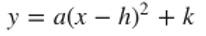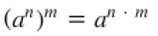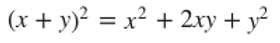###### Upcoming Events
Essential SAT Math Formulas You Need To Know

Now that you have the foundational formulas given to you for the SAT math section, 7EDU is here to bring you the top 10 SAT math formulas that you will need to know to succeed and they are not given to you on the test.

As mentioned in yesterday's article, after the new SAT was implemented, geometry now makes less up than ten percent of the exam math questions. To provide students with the latest formulas that you will absolutely need to be successful on your exam, the theorems provided to you in today's blog will focus primarily on arithmetic and algebra.

Bookmark this article so you can refer back to it easily when you are studying and practicing how to apply the formulas strategically.

By planning beforehand and committing the following concepts and formulas to your memory, you will save time that you can use on other portions of the examination but also be properly prepared for mathematical questions that cannot be answered with the general formulas provided on the SAT test.

Interested in enhancing your college resume? Maybe try a writing competition ✏️ or even a STEM contest 🧬.

Without further ado, let's jump into it!## UC Berkeley AI Program

that 7EDU is partnering up with, see the flyer at the end of this article!

### Every Friday from 5:30 - 6:00 pm PST 🎙 Consulting Jun

Slope-Intercept Form of a Line• The m in the equation refers to the slope of the equation

• The b in the equation refers to the y-value of the y-intercept

• For example, if we have the equation y = 3x + 9: the slope is 3 and the y-intercept is (0,9)

Typically on the SAT, you will find that they have provided you an equation in a different format. Be sure to rewrite the equation into the slope-intercept form of a line so you can correctly interpret what the slope or intercept is.

Slope Formula

Given two points,Find the slope of the line that connects them. You will need to know the following formula in order to successfully find the slope that connects the two points:Note that the slope of a line is calculated by:Vertex or Quadratic Form of a Parabola

What the vertex form of a parabola should look like:And in case you were familiar with the factored form of the quadratic:When you are taking the SAT math section, you will need to be able to distinguish the vertex form and be able to transform quadratics to the vertex on the SAT.

The values of h and k refer to the coordinates of the vertex, (h, k).The quadratic formula will be beneficial to memorize as it will help you find the roots of a quadratic equation (a parabola) if you cannot simply factor it. If your parabola equation is in the factored form mentioned above in "Vertex or Quadratic Form of a Parabola," then you will only need to plug the coefficients and constants into the quadratic formula.

Distance Formula

Given two points,Find the distance between the two points. You will need to know the following formula in order to successfully find the distance between the two points:The distance formula is derived from the Pythagorean Theorem and is helpful for figuring out the distance between two points. This formula is efficient but isn't absolutely crucial, as you can take a different approach if you utilize the Pythagorean Theorem:

2. Create a right triangle from the points

3. Solve for the distance through the right triangle's hypotenuse

Exponent Rules MultiplicationWhen you have the same base numbers raised to different powers and are being multiplied together, you will add the exponents.

Exponent Rules Power Raised to a PowerWhen you are raising a power to another power, multiply the exponents.

Exponent Rules DivisionWhen you have the same base number raised to different powers and are being divided, you will subtract the exponents.

Binomial Product 1 Difference of SquaresBy strategically recognizing the binomial products and factoring them on the no-calculator section of the SAT, you will save time for other questions. Convert the binomial products from their factored forms to the expanded version immediately.

Binomial Product 2 Perfect Squares Trinomial (Positive or Negative)Another great formula to get familiar with is the perfect square trinomial. Whether the quantity involves addition or subtraction, you will save time if you are able to convert the binomial products from their factored forms to the expanded.

Complex ConjugateWhile taking the SAT test, you can expect to see at least one math question where you will need to control imaginary numbers. By using the complex conjugate formula, you will be able to remove the imaginary portion of the complex number and find the real number.

Here is a 9-minute video that summarizes need-to-know SAT math formulas:7EDU Impact Academy 7 July, 2021